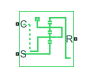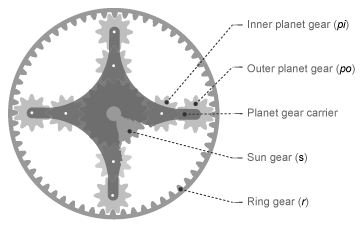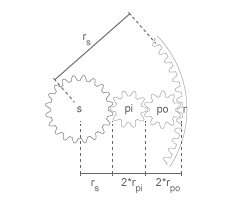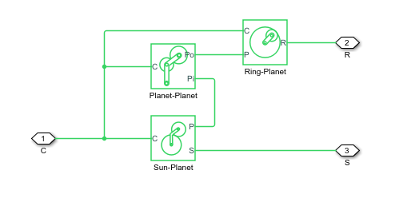# Double-Pinion Planetary Gear

Planetary gear train with two meshed planet gear sets

•Libraries:
Simscape / Driveline / Gears

## Description

The Double-Pinion Planetary Gear block represents a planetary gear train with two meshed planet gear sets between the sun gear and the ring gear. A single carrier holds the two planet gear sets at different radii from the sun gear centerline, while allowing the individual gears to rotate with respect to each other. The gear model includes power losses due to friction between meshing gear teeth and viscous damping of the spinning gear shafts.Structurally, the double-pinion planetary gear resembles a Ravigneaux gear without its second, large, sun gear. The inner planet gears mesh with the sun gear and the outer planet gears mesh with the ring gear. Because it contains two planet gear sets, the double-pinion planetary gear reverses the relative rotation directions of the ring and sun gears.

The teeth ratio of a meshed gear pair fixes the relative angular velocities of the two gears in that pair. In the Double-Pinion Planetary Gear block, you can specify the gear teeth ratios between the ring and sun gears and the outer planet and inner planet gears. A geometric constraint fixes the remaining teeth ratios—the ring gear to the outer planet gear and the inner planet gear to the sun gear. This constrains the ring gear radius to the sum of the sun gear radius and the inner and outer planet gear diameters.

`${r}_{r}={r}_{s}+2\cdot {r}_{pi}+2\cdot {r}_{po},$`

where:

• rr is the ring gear radius.

• rs is the sun gear radius.

• rpi is the inner planet gear radius.

• rpo is the outer planet gear radius.The ring to outer planet gear teeth ratio is

`$\frac{{r}_{r}}{{r}_{po}}=2\cdot \frac{\frac{{r}_{r}}{{r}_{s}}}{\left(\frac{{r}_{r}}{{r}_{s}}-1\right)}\cdot \frac{\left(\frac{{r}_{po}}{{r}_{pi}}+1\right)}{\frac{{r}_{po}}{{r}_{pi}}}.$`

The inner planet-sun teeth ratio is

`$\frac{{r}_{pi}}{{r}_{s}}=\frac{\left(\frac{{r}_{r}}{{r}_{s}}-1\right)}{2\left(\frac{{r}_{po}}{{r}_{pi}}+1\right)}.$`

The Double-Pinion Planetary Gear block is a composite component. It contains three underlying blocks—Ring-Planet, Planet-Planet, and Sun-Planet—connected as shown in the figure. Each block connects to a separate drive shaft through a rotational conserving port.### Thermal Model

You can model the effects of heat flow and temperature change by enabling the optional thermal port. To enable the port, set Friction model to ```Temperature-dependent efficiency```.

### Assumptions and Limitations

• Gear inertia is assumed to be negligible.

• Gears are treated as rigid components.

## Ports

### Conserving

expand all

Rotational mechanical conserving port associated with the planet gear carrier.

Rotational mechanical conserving port associated with the ring gear.

Rotational mechanical conserving port associated with the sun gear.

Thermal conserving port associated with heat flow. Heat flow affects the power transmission efficiency by altering the gear temperatures.

#### Dependencies

To enable this port, set Friction model to `Temperature-dependent efficiency`.

## Parameters

expand all

### Main

Fixed ratio of the ring gear to the sun gear rotations as defined by the number of planet gear teeth divided by the number of sun gear teeth.

Fixed ratio of the outer planet gear to the inner planet gear rotations as defined by the number of planet gear teeth divided by the number of sun gear teeth.

### Meshing Losses

Friction model for the block:

• `No meshing losses - Suitable for HIL simulation` — Gear meshing is ideal.

• `Constant efficiency` — Transfer of torque between the gear wheel pairs is reduced by a constant efficiency, η, such that 0 < η ≤ 1.

• `Temperature-dependent efficiency` — Transfer of torque between the gear wheel pairs is defined by the table lookup based on the temperature.

Vector of torque transfer efficiencies, [ηSP, ηRP, ηRPP], for sun-planet, and ring-planet, and planet-planet gear wheel pair meshings, respectively. The vector elements must be in the interval (0,1].

#### Dependencies

To enable this parameter, set Friction model to `Constant efficiency`.

Vector of temperatures used to construct a 1-D temperature-efficiency lookup table. The vector elements must increase from left to right.

#### Dependencies

To enable this parameter, set Friction model to `Temperature-dependent efficiency`.

Vector of output-to-input power ratios that describe the power flow from the sun gear to the inner planet gears, ηSP. The block uses the values to construct a 1-D temperature-efficiency lookup table.

Each element is an efficiency that relates to a temperature in the Temperature vector. The length of the vector must be equal to the length of the Temperature vector. Each element in the vector must be in the range (0,1].

#### Dependencies

To enable this parameter, set Friction model to ```Temperature-dependent efficiency```.

Vector of output-to-input power ratios that describe the power flow from the ring gear to the outer planet gears, ηRP. The block uses the values to construct a 1-D temperature-efficiency lookup table.

Each element is an efficiency that relates to a temperature in the Temperature vector. The length of the vector must be equal to the length of the Temperature vector. Each element in the vector must be in the range (0,1].

#### Dependencies

To enable this parameter, set Friction model to ```Temperature-dependent efficiency```.

Vector of output-to-input power ratios that describe the power flow from the inner planet gear to the outer planet gear, ηPP. The block uses the values to construct a 1-D temperature-efficiency lookup table.

Each element is an efficiency that relates to a temperature in the Temperature vector. The length of the vector must be equal to the length of the Temperature vector. Each element in the vector must be in the range (0,1].

#### Dependencies

To enable this parameter, set Friction model to ```Temperature-dependent efficiency```.

Vector of power thresholds above which the full efficiency factors apply. Enter the thresholds in the order sun-carrier, ring-carrier, planet-carrier.

When you set Friction model to `Constant efficiency`, the block lowers the efficiency losses to zero when no power is transmitted. When you set Friction model to `Temperature-dependent efficiency`, the block smooths the efficiency factors between zero when at rest and the values provided by the temperature-efficiency lookup tables at the power thresholds.

The power threshold should be lower than the expected power transmitted during simulation. Higher values may cause the block to underestimate efficiency losses. Very low values may raise the computational cost.

#### Dependencies

To enable the parameter, set Friction model to `Constant efficiency` or ```Temperature-dependent efficiency```.

### Viscous Losses

Vector of viscous friction coefficients, [μS μR μP], for the sun-carrier, ring-carrier, and planet-carrier gear motions, respectively.

### Inertia

Inertia model for the block:

• `Off` — Model gear inertia.

• `On` — Neglect gear inertia.

Moment of inertia of the inner planet gears. This value must be positive.

#### Dependencies

To enable this paramter, set Inertia to `On`.

Moment of inertia of the outer planet gears. This value must be positive.

#### Dependencies

Tho enable this parameter, set Inertia to `On`.

### Thermal Port

To enable these settings, set Friction model to `Temperature-dependent efficiency`.

Thermal energy required to change the component temperature by a single temperature unit. The greater the thermal mass, the more resistant the component is to temperature change.

#### Dependencies

To enable this parameter, set Friction model to ```Temperature-dependent efficiency```.

Block temperature at the start of simulation. The initial temperature sets the initial component efficiencies according to their respective efficiency vectors.

#### Dependencies

To enable this parameter, set Friction model to `Temperature-dependent efficiency`.

expand all

## Version History

Introduced in R2013b